NCERT Solutions for Class 8 Maths Chapter 3 Understanding Quadrilaterals Ex 3.1 are part of NCERT Solutions for Class 8 Maths. Here we have given NCERT Solutions for Class 8 Maths Chapter 3 Understanding Quadrilaterals Ex 3.1.

 Board CBSE Textbook NCERT Class Class 8 Subject Maths Chapter Chapter 3 Chapter Name Understanding Quadrilaterals Exercise Ex 3.1 Number of Questions Solved 7 Category NCERT Solutions

## NCERT Solutions for Class 8 Maths Chapter 3 Understanding Quadrilaterals Ex 3.1

Question 1.
Given here are some figures :Classify each of them on the basis of the following :
(a) Simple curve
(b) Simple closed curve
(c) Polygon
(d) Convex polygon
(e) Concave polygon
Solution.
(a) ⟷ 1, 2, 5, 6, 7
(b) ⟷ 1, 2, 5, 6, 7

Question 2.
How many diagonals does each of the following have?
(b) A regular hexagon
(c) A triangle.
Solution.
(a) → 2
(b) → 9
(c) → 0

Question 3.
What is the sum of the measures of the angles of a convex quadrilateral? Will this property hold if the quadrilateral is not convex? (Make a non-convex quadrilateral and j try!)
Solution.
The sum of the measures of the angles of a convex quadrilateral is 360°.
Yes! this property will hold if the; quadrilateral is not convex.
If the quadrilateral is not convex, then it will be concave.
Split the concave quadrilateral ABCD into two triangles ABD and CBD by joining BD.Question 4.
Examine the table. (Each figure is divided into triangles and the sum of the angles deduced from that.)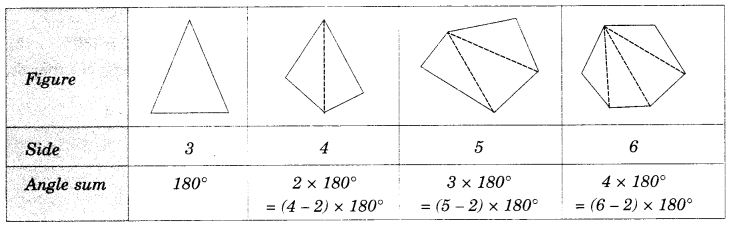What can you say about the angle sum of a convex polygon with number of sides ?
(a) 7
(b) 8
(c) 10
(d) n
Solution.
(a) 7
Angle sum = $$\left( 7-2 \right) \times { 180 }^{ \circ }$$
= $$5\times { 180 }^{ \circ }={ 900 }^{ \circ }$$

(b) 8
Angle sum = $$\left( 8-2 \right) \times { 180 }^{ \circ }$$
= $$6\times { 180 }^{ \circ }={ 1080 }^{ \circ }$$

(c) 10
Angle sum = $$\left( 10-2 \right) \times { 180 }^{ \circ }$$
= $$8\times { 180 }^{ \circ }={ 1440 }^{ \circ }$$

(d) n
Angle sum = $$\left( n-2 \right) \times { 180 }^{ \circ }$$

Question 5.
What is a regular polygon? State the name of a regular polygon of
(i) 3 slides
(ii) 4 slides
(iii) 6 slides
Solution.
A polygon, which is both ‘equilateral’ and ‘equiangular’, is called a regular polygon.
(i) 3 slides
The name of the regular polygon of 3 slides is an equilateral triangle.
(ii) 4 slides
The name of the regular polygon of 4 slides is square
(iii) 6 slides
The name of the regular polygon of 6 slides is a regular hexagon

Question 6.
Find the angle measure x in the following figures.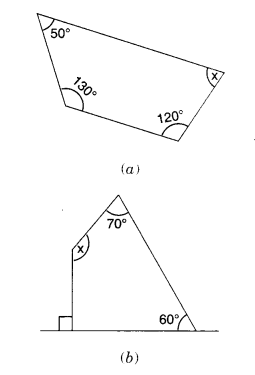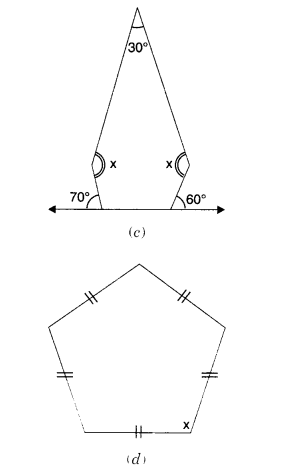Solution.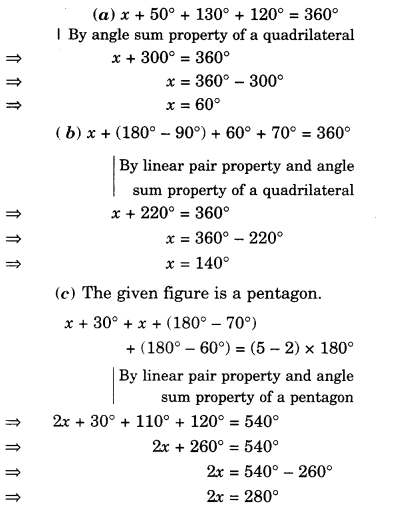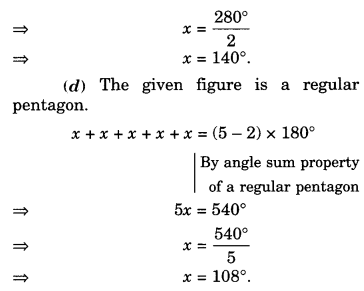Question 7.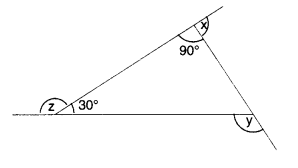(a) Find x+y+z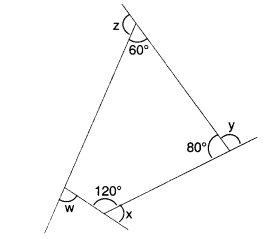(b) Find x+y+z+w.
Solution.We hope the NCERT Solutions for Class 8 Maths Chapter 3 Understanding Quadrilaterals Ex 3.1 help you. If you have any query regarding NCERT Solutions for Class 8 Maths Chapter 3 Understanding Quadrilaterals Ex 3.1, drop a comment below and we will get back to you at the earliest.